# StandardDeviation

Calculates the empirical standard deviation of its input signal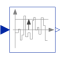# Information

This information is part of the Modelica Standard Library maintained by the Modelica Association.

This block calculates the standard deviation of its input signal. The standard deviation is the square root of the signal's variance:

`y = sqrt( variance(u) )`

The Variance block is used to calculate variance(u).

The parameter t_eps is used to guard against division by zero (the computation of the standard deviation starts at <simulation start time> + t_eps and before that time instant y = 0).

This block is demonstrated in the examples UniformNoiseProperties and NormalNoiseProperties.

# Parameters (1)

t_eps Value: 1e-7 Type: Time (s) Description: Standard deviation calculation starts at startTime + t_eps

# Connectors (2)

u y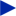Type: RealInput Description: Noisy input signal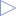Type: RealOutput Description: Standard deviation of the input signal

# Components (2)

variance sqrt1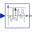Type: Variance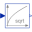Type: Sqrt

# Used in Examples (2)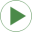UniformNoiseProperties Modelica.Blocks.Examples.NoiseExamples Demonstrates the computation of properties for uniformally distributed noiseNormalNoiseProperties Modelica.Blocks.Examples.NoiseExamples Demonstrates the computation of properties for normally distributed noise